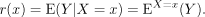# 3.1. Definition

Let (X, Y) be a pair of random variables defined on the probability spacein which only X is observed. We wish to know what information X carries about Y: this is the filtering problem defined in Chapter 1.

This problem may be formalized in the following way: supposing Y to be real and square integrable, construct a real random variable of the form r(X) that gives the best possible approximation of Y with respect to the quadratic error, i.e. E[(Yr(X))2] being minimal.

If we identify the random variables with their P-equivalence classes, we deduce that r(X) exists and is unique, since it is the orthogonal projection (in the Hilbert space L2 (P)) of Y on the closed vector subspace L2 (P) constituted by the real random variables of the form h(X) and such that E[(h(X))2] < +∞.

From Doob’s lemma, the real random variables of the form h(X) are those that are measurable with respect to the σ-algebra generated by X. We say that r(X) is the conditional expectation of Y with respect to the σ-algebra generated by X (or with respect to X), and that r is the regression of Y on X. We write:The above equation leads us to the following definition.

DEFINITION 3.1.– Let be a probability space and let be a sub-σ-algebra of . We call the orthogonal ...

Get Mathematical Statistics and Stochastic Processes now with the O’Reilly learning platform.

O’Reilly members experience books, live events, courses curated by job role, and more from O’Reilly and nearly 200 top publishers.